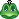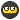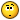## Recommended Posts

I tried to solve the math needed to find a puzzle for a cache. The solution will be the coordinates. I did the math, but I can't put it together to get the coordinates. The equations were in bracket boxes, like this "[A - B][b+3]" etc. This does not seem to result in any sort of coordinates. I can find nothing online about multiple groups of operations. Do I multiply, ignore zeros, or what??A "checksum" is mentioned, but I have no clue how to use it.The solution, minus the boxes, won't fit into the geocheck answer fields either!

Help is requested!

Perhaps post the link to the cache? Kind of hard to help without seeing the puzzle itself.

Perhaps post the link to the cache? Kind of hard to help without seeing the puzzle itself.

This one: [Link Removed by Moderator]

Edited by Keystone
Ask for general help, not help in solving specific puzzles. Thanks!

I tried to solve the math needed to find a puzzle for a cache. The solution will be the coordinates. I did the math, but I can't put it together to get the coordinates. The equations were in bracket boxes, like this "[A - B][b+3]" etc. This does not seem to result in any sort of coordinates. I can find nothing online about multiple groups of operations. Do I multiply, ignore zeros, or what??A "checksum" is mentioned, but I have no clue how to use it.The solution, minus the boxes, won't fit into the geocheck answer fields either!

Help is requested!

Have you asked the Cache Owner? That person probably knows the solution.I tried to solve the math needed to find a puzzle for a cache. The solution will be the coordinates. I did the math, but I can't put it together to get the coordinates. The equations were in bracket boxes, like this "[A - B][b+3]" etc. This does not seem to result in any sort of coordinates. I can find nothing online about multiple groups of operations. Do I multiply, ignore zeros, or what??A "checksum" is mentioned, but I have no clue how to use it.The solution, minus the boxes, won't fit into the geocheck answer fields either!

Help is requested!

Have you asked the Cache Owner? That person probably knows the solution.I don't want the solution, I want to know how to figure it out.

I tried to solve the math needed to find a puzzle for a cache. The solution will be the coordinates. I did the math, but I can't put it together to get the coordinates. The equations were in bracket boxes, like this "[A - B][b+3]" etc. This does not seem to result in any sort of coordinates. I can find nothing online about multiple groups of operations. Do I multiply, ignore zeros, or what??A "checksum" is mentioned, but I have no clue how to use it.The solution, minus the boxes, won't fit into the geocheck answer fields either!

Help is requested!

Each set of brackets results in a single digit. So in your example, you have to solve for the value of A and B. Usually, you will observe something on a sign that tells you what each letter is. So if you determine that A=7 and B=3, you can solve what's inside the brackets and end up with 13 in this case.

I tried to solve the math needed to find a puzzle for a cache. The solution will be the coordinates. I did the math, but I can't put it together to get the coordinates. The equations were in bracket boxes, like this "[A - B][b+3]" etc. This does not seem to result in any sort of coordinates. I can find nothing online about multiple groups of operations. Do I multiply, ignore zeros, or what??A "checksum" is mentioned, but I have no clue how to use it.The solution, minus the boxes, won't fit into the geocheck answer fields either!

Help is requested!

Each set of brackets results in a single digit. So in your example, you have to solve for the value of A and B. Usually, you will observe something on a sign that tells you what each letter is. So if you determine that A=7 and B=3, you can solve what's inside the brackets and end up with 13 in this case.

The example was not relative. A = a 3-digit number. Thanks for trying, though.

If the numbers are 3682 then A=3 B=6 C=8 D=2. Therefore [C-B][A+D][C-A] would be [8-6][3+2][8-3]....

The geochecker will check the final coords, not each number in the brackets.

Hope this helps.

If the numbers are 3682 then A=3 B=6 C=8 D=2. Therefore [C-B][A+D][C-A] would be [8-6][3+2][8-3]....

The geochecker will check the final coords, not each number in the brackets.

Hope this helps.

Sorry, your method still gives me the same results. Removing the brackets gives me a mess. Someone posted a good method, but when I refreshed, it went away.Ask the cache owner for clarification.

Ask the cache owner for clarification.

Will do. Thanks.

## Join the conversation

You can post now and register later. If you have an account, sign in now to post with your account.
Note: Your post will require moderator approval before it will be visible.×   Pasted as rich text.   Paste as plain text instead

Only 75 emoji are allowed.